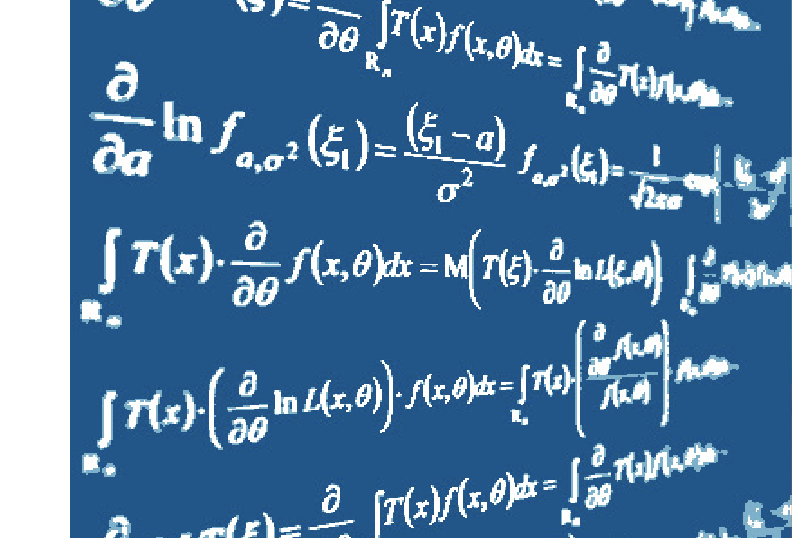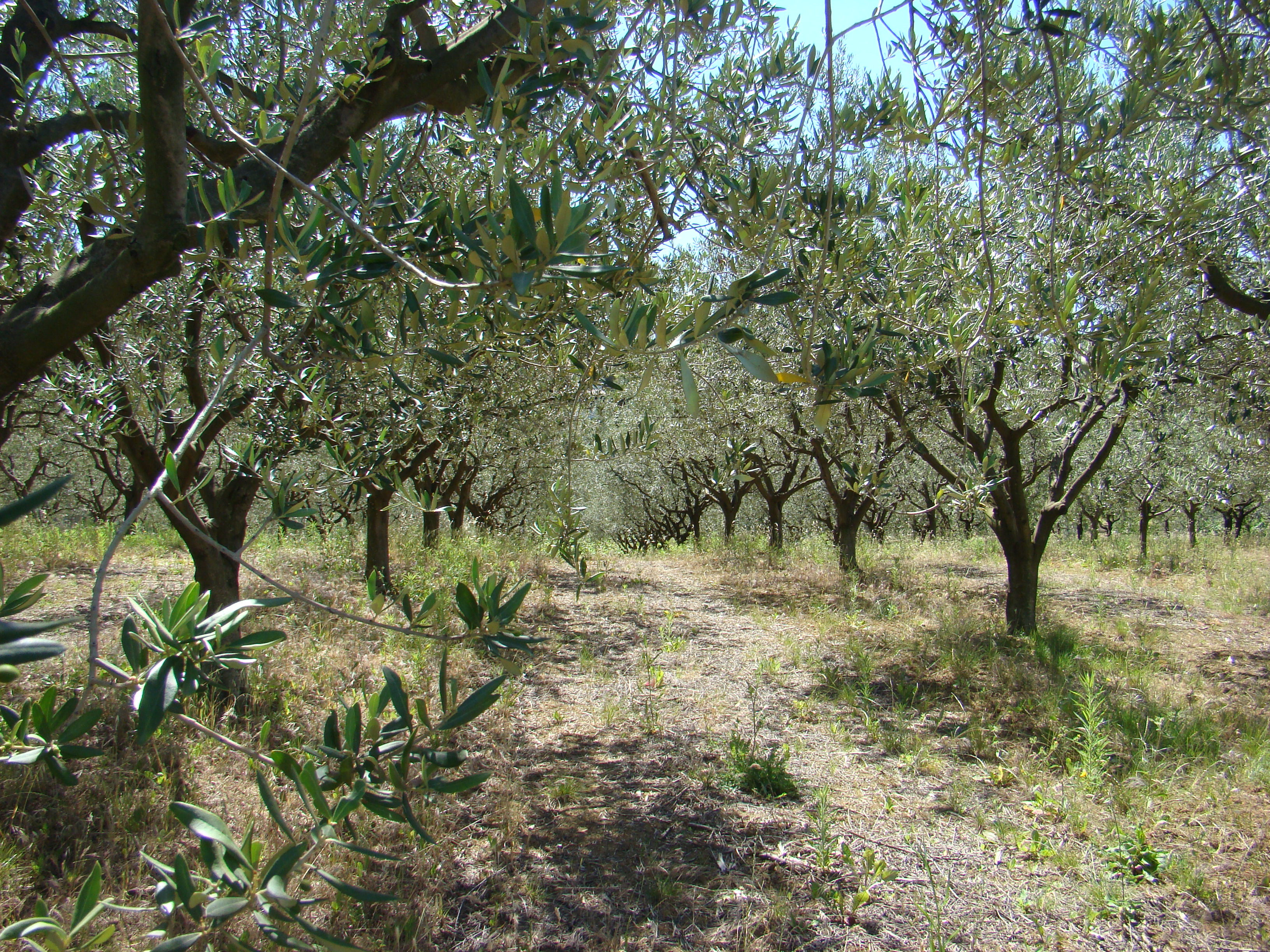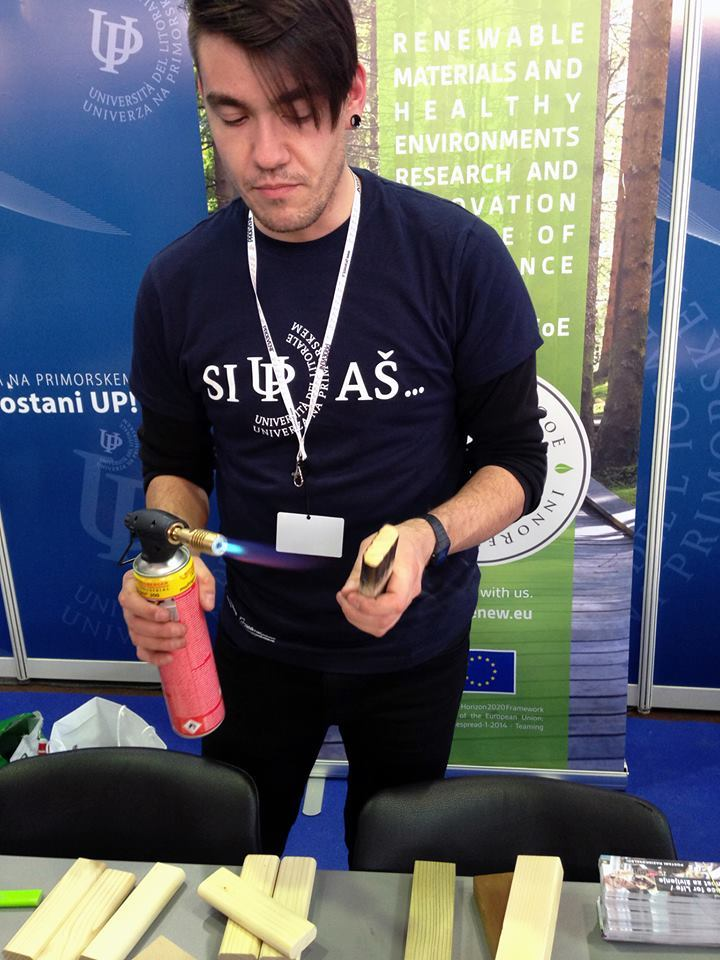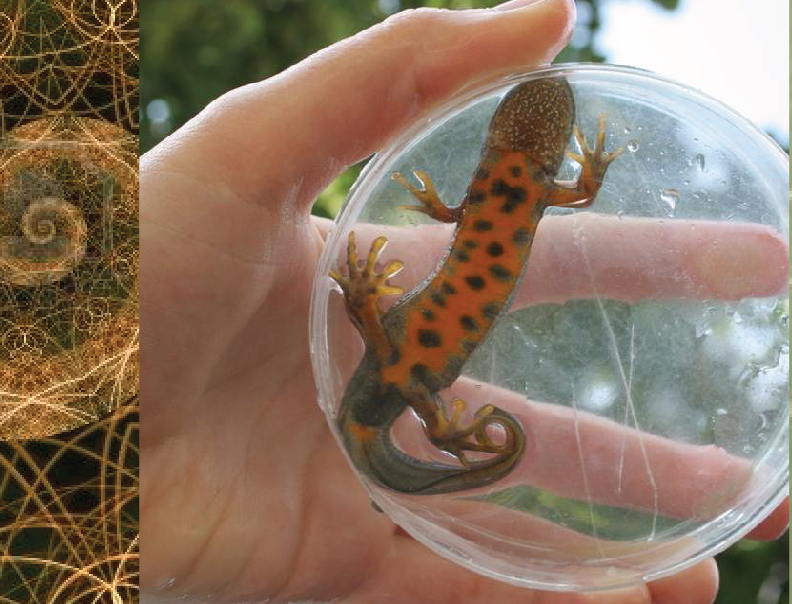Univerza na Primorskem Fakulteta za matematiko, naravoslovje in informacijske tehnologijeSI | EN
natisni

Orel Marko, University of Primorska, Slovenia.

Title: Graph homomorphisms and applications

Abstract: Preserver problems is a research area in matrix theory, where a typical problem demands a characterization of all maps between two sets of matrices that satisfy some additional property (e.g. they preserve some relation, function or a subset). It often turns out that the studied maps are all bijective. This phenomenon can be sometimes translated in the language of graph theory as saying that all endomorphisms of a certain graph are automorphisms. Such graphs are called cores and are being widely studied in the last two decades. In the talk I will present few results from preserver problems, where tools from graph theory were applied to solve the problem. For large classes of graphs it is known that a graph is either a core or its chromatic number equals its clique number. Sometimes it is difficult to decide which of the two possibilities occurs. In the talk I will present a graph, where this dilemma is essentially equivalent to a long standing open problem in finite geometry. The talk will end with another open problem with a statement that does not require preknowledge to understand it and may be appropriate for PhD students.